• 主要介绍了Laravel框架实现model层的增删改查(CURD)操作,结合实例形式分析了Laravel框架模型model层进行数据库的增删改查操作具体实现技巧,需要的朋友可以参考下
• Document {{csrf_field()}} 用户名 手机号 记录学习laravel8.6实现增删改查，希望对初学习的你有所帮助 1.添加表单 2.展示表单 Document id 用户名 手机号 操作 @foreach($data as$v) {{$v->id}} {{$v->username}} ...
<!DOCTYPE html>
<html lang="en">
<meta charset="UTF-8">
<meta http-equiv="X-UA-Compatible" content="IE=edge">
<meta name="viewport" content="width=device-width, initial-scale=1.0">
<title>Document</title>
<body>
{{csrf_field()}}
手机号 <input type="tel" name="phone" id=""><br>
<input type="submit" value="添加">
</form>
</body>
</html>
记录学习laravel8.6实现增删改查，希望对初学习的你有所帮助
1.添加表单
2.展示表单
<!DOCTYPE html>
<html lang="en">
<meta charset="UTF-8">
<meta http-equiv="X-UA-Compatible" content="IE=edge">
<meta name="viewport" content="width=device-width, initial-scale=1.0">
<title>Document</title>
<body>
<table>
<tr>
<td>id</td>
<td>用户名</td>
<td>手机号</td>
<td>操作</td>
</tr>
@foreach($data as$v)
<tr>
<td>{{$v->id}}</td> <td>{{$v->username}}</td>
<td>{{$v->phone}}</td> <td> <a href="delete?id={{$v->id}}">删除</a>||
<a href="edit?id={{$v->id}}">编辑</a> </td> </tr> @endforeach </table> </body> </html> 3.编辑页面 <!DOCTYPE html> <html lang="en"> <head> <meta charset="UTF-8"> <meta http-equiv="X-UA-Compatible" content="IE=edge"> <meta name="viewport" content="width=device-width, initial-scale=1.0"> <title>编辑</title> </head> <body> <form action="update" method="post"> <input type="hidden" name="id" value="{{$info->id}}">
{{csrf_field()}}
用户名 <input type="text" name="username" value="{{$info->username}}"><br> 手机号 <input type="tel" name="phone" value="{{$info->phone}}"><br>
<input type="submit" value="编辑">
</form>
</body>
</html>
4.使用查询构造器实现的
控制器方法
<?php
namespace App\Http\Controllers;
use Illuminate\Routing\Controller;
use Illuminate\Http\Request;
// 引入DB类
class TestController extends Controller{
public function index(){
return view("test/index");
}
// 添加 推荐使用的方式
public function add(Request $request){ // 接收表单传过来的数据$data['username'] = $request['username'];$data['phone'] = $request['phone'];$data['created_ad'] = date("Y-m-d h:i:s",time());
// 执行添加操作
$res = DB::table('member')->insert($data);
// dd($res);返回true 表示添加成功 false表示失败 if($res){
// 添加成功跳转至列表页面
return redirect('show');
}else{
// 添加失败跳转至添加页面
return redirect('index');
}
}
// 列表展示
public function show()
{
$data = DB::table("member")->get(); return view("test/show",["data"=>$data]);
}
// 删除
public function delete(Request $request) { //接收id$where['id'] =$request['id'];$res = DB::table('member')->where($where)->delete(); //dd($res);返回1 表示删除成功 0表示未做删除
if($res){ // 删除成功跳转至列表展示 return redirect('show'); }else{ return redirect('show'); } } //编辑 public function edit(Request$request)
{
# 接收id
$where['id'] =$request['id'];
$info = DB::table('member')->where($where)->first();
return view("test/edit",["info"=>$info]); } // 修改 public function update(Request$request)
{
# 接收id
$where['id'] =$request['id'];
# 接收新值
$data['username']=$request['username'];
$data['phone']=$request['phone'];
$res = DB::table('member')->where($where)->update($data); // dd($res);
if($res === 1){ return redirect('show'); }else{ return redirect('show'); } } } ?>  5.路由 路由设置这里可以看我上一篇路由设置 Route::get('indexs','TestController@index'); // 添加方法 Route::post('add','TestController@add'); // 列表展示 Route::get('show','TestController@show'); //删除 Route::get('delete','TestController@delete'); //编辑 Route::get('edit','TestController@edit'); Route::post('update','TestController@update');  6.表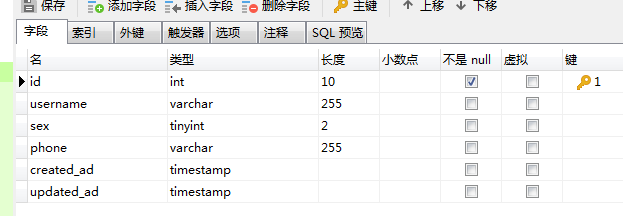原创不易，关注，点赞支持，谢谢老铁  展开全文html html5 javascript • 解压一个laravel到根目录下，然后用命令行访问到laravel下， 在命令行上面生成控制器，模型，迁移文件 php artisan make:controller TestController php artisan make:model Test ... 在database/migrations/你生成的... 解压一个laravel到根目录下，然后用命令行访问到laravel下， 在命令行上面生成控制器，模型，迁移文件 php artisan make:controller TestController php artisan make:model Test php artisan make:migration create_test_table  在database/migrations/你生成的新的迁移文件中创建表字段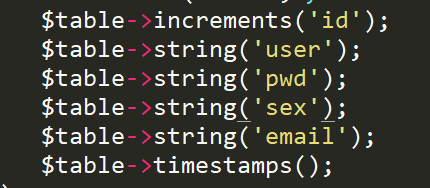在config/databases.php中配置数据库 在.env下配置数据库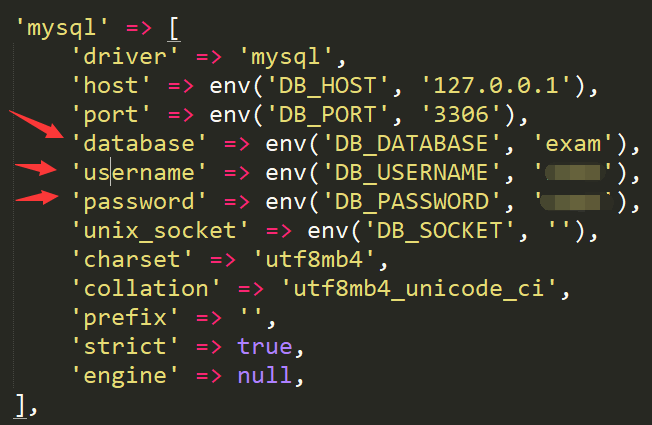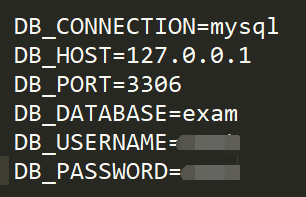在打开命令行执行迁移文件 php artisan migrate  在app/Test下模型增加字段名和数据库名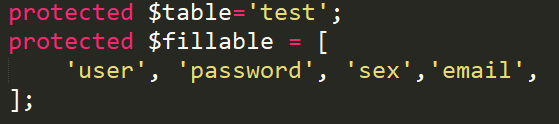现在进入控制器添加 public function adds(Request$request){
$model=new Test();$model->user=$request->user;$model->pwd=$request->pwd;$model->sex=$request->sex;$model->email=$request->email;$data=$model->save(); if($data){
return redirect('show');
}else{
return json_encode(['code'=>'500','msg'=>'添加失败']);
}
}

展示
public function show(){
$data=Test::all(); return view('test.show',compact('data')); }  修改 public function upd(){$id=$_GET['id'];$data=Test::findOrFail($id); return view('test.upd',compact('data')); }  public function upda(Request$request){
$id=$_POST['id'];
$model=Test::findOrFail($id);
$model->update([ 'user'=>$request->user,
'pwd'=>$request->pwd, 'sex'=>$request->sex,
'email'=>$request->email, ]); return redirect('show'); }  删除 public function del(){$id=$_GET['id'];$data=Test::findOrFail($id);$data=$data->delete(); if($data){
return redirect('show');
}else{
return redirect('show');
}
}

展开全文• Laravel操作数据库增删改查的三种方法 第一种：数据库操作DB facade(原始查找)： 增：$bool = DB::insert('insert into student (name,age) value (?,?)',['Bob','20']); var_dump($bool); //把id=2的数据删除掉 ...
Laravel操作数据库增删改查的三种方法
增：$bool = DB::insert('insert into student (name,age) value (?,?)',['Bob','20']); var_dump($bool);
//把id=2的数据删除掉

删：$num = DB::delect('delect from student where id = ?',); var_dump($num);
//把age和name这两个字段的数据

改: $num = DB::update('update student set age = ? where name =?',['23','Bob']); var_dump($num);
//进行有条件的查询，查询出id>2的数据

查：$student = DB::select('select * from student where id > ?',); var_dump($student);

第二种：查找构造器(常用写法)
查询构造器的简介：
（1）Lavavel查询构造器提供了方便的流畅的接口，用来建立及执行数据库查找的语法
（2）使用PDO参数绑定，以保护应用程序免于SQL注入（因为它的写法免去了SQL语句），因此传入的参数不需要额外的转义特殊字符
（3）基本上满足所有的数据库操作，而且在所有支持的数据库系统上都可以执行
--------------------------------------------------------------------------------------------
增：$bool = DB::table('student')->insert(['name'=>'Alice','age'=>'20']); var_dump($bool);

//想要获取添加的这条数据的自增id
$id = DB::table('student')->insert(['name'=>'Amanda','age'=>'26']); var_dump($id);

//想要插入多条数据(其实就是二维数组)
$bool = DB::table('student')->insert([ ['name'=>'Alice','age'=>'25'], ['name'=>'Amanda','age'=>'26'], ]); var_dump($bool);
--------------------------------------------------------------------------------------------
删：//删有两种： 1.delect  2.truncate
1.delect:
$num = DB::table('student') ->where('id',3) ->delect();$var_dump($num); 1.1带条件的删除(删除id>=8的数据)： DB::table('student') ->where('id','>=',8) ->delect(); var_dump(); 2.truncate：整表删除，谨慎使用！！ DB::table('student')->truncate(); -------------------------------------------------------------------------------------------- 改: //修改有3种：1.修改为指定的内容； 2.自增和自减 3.自增自减带条件 1.修改为指定的内容(把id为7的数据里面age这个字段的数值修改为30):$num = DB::table('student')
->where('id',7)
->update(['age'=>'30']);
var_dump($num); 2.自增和自减： //自增： //inscrment()函数:数据库指定的字段数值就会自增1$num = DB::table('student')->inscrement('age');
var_dump($num); //自减： //decrement()函数:自减,数据库指定的字段的数值就会自减1$num = DB::table('student')->decrement('age');
var_dump($num); 3.1自增自减带条件(每条age字段的数据自减3):$num = DB::table()->inscrement('age',3);
var_dump($num); 或者是带以下这样的条件的： 3.2将数据库里id为3的age字段自减4：$num = DB::table('student')
->where('id',3),
->decrement('age',4);
var_dump($num); 3.3自增和自减的时候其他条件发生变化：$num = DB::table('student')
->where('id',3),
->decrement('age',4,['name'=>'Boo']);
var_dump($num); -------------------------------------------------------------------------------------------- 查询有以下7种方法： 1.get() | 2.first() | 3.where() | 4.pluck() | 5.lists() | 6.select() | 7.chunk() 查：//1.get()获取表中所有数据$students = DB::table('student')->get();
dd($students); //2.first()获取表中的第一条数据$students = DB::table('student')->first();
dd($students); //3.where()有条件的查询$students = DB::table('student')
->where('id','>=',5);
->get();
var_dump($students); //3.1给where加多个条件(查询id>=5 且 age>=27的数据）$students = DB::table('student')
->where('id >= ? and age >= ?',[5,27])
-get();
var_dump($students); //4.pluck()返回结果集中指定的字段$name = DB::table('student')->pluck('name');
dd($name); //5.lists()返回结果集中指定的字段(结果和pluck()函数的一样）$name = DB::table('student')->pluck('name');
dd($name); //5.1 lists()还可以设置指定的键作为下标$name = DB::table('student')->lists('name','id');
dd($name); //6.select()可以查询字段$students = DB::table('student')
->select('name','age')
->get();
dd($students); //7.chunk()分段式的查询 DB::table('student')->chunk(2,function($students) {
var_dump($students); } //7.1 假如只需要chunk查询一次就停止查询 DB::table('student')->chunk(2,function($students) {
var_dump($students); return false; }  第三种：Eloquent ORM Eloquent ORM的简介 laravel所自带的Eloquent ORM是一个非常优美简洁的ActiveRecord实现， 用来实现数据库的操作他的每个数据的表都有相对应的模型(model)用于数据表的交互。 -------------------------------------------------------------------------------------------- //Eloquent ORM新增数据的两种方法： //1.过模型新增数据(涉及到自定义时间戳) //2.用模型的Create方法新增数据(涉及到批量赋值) 增： NO.1通过模型新增数据： 第一步根据数据表(student.sql),新建一个数据表的模型(Student.php) 在创建对应的StudentController控制器里面使用模型新增数据(注意时间戳的问题) public function orm2() { //使用模型新增数据$student = new Student();
$student -> name = 'xiaohei1';$student -> age = 2;
$bool =$student -> save();
dd($bool); } 在模型里指定数据表、指定id、自定义时间戳。 //指定数据库 protected$stable = 'student';
//指定id
protected $primaryKey = 'id'; //不想要时间戳,设置为false这样返回的数据就没有时间戳 / 要时间戳则为true; public$timestamps = false;
//getDateFormat()这个函数会返回一个时间
protected function getDateFormat()
{
return time();
}

NO.2 用模型的Create方法新增数据(涉及到批量赋值)
在StudentController里面
$student = Student::create( ['name'=>'xiaoqiang','age'=>4] ); dd($student);
接下来就是打开Student.php模型去添加批量赋值的属性
//允许批量赋值
//允许这两个字段批量赋值
protected $fillable = ['name','age']; -------------------------------------------------------------------------------------------- 删： 1.Eloquent ORM删除数据的三种方法： 1.通过模型进行删除 2.通过主键值进行删除 3.根据指定的条件进行删除 1.通过模型进行删除 //删除id为13的这一条数据$student = Student::find(13);
$bool =$student->delect();
var_dump($bool); 2.通过主键进行删除 //删除主键(id)为12的数据$num = Student::destroy(ids:12);
var_dump($num); 或者 //输出主键(id)为11和10的数据$num = Student::destroy(ids:11,10);
var_dump($num); 或者 //输出主键(id)为9和18的数据$num = Student::destroy([9,8]);
var_dump($num); 3.根据指定的条件进行删除 //删除id>6的数据$num = Student::where('id','>',6)->delecte();
var_dump($num); -------------------------------------------------------------------------------------------- 改: 1.Eloquent ORM更新数据的两种方法： //1.通过模型更新数据 2.结合查询查询语句批量更新数据 //1.通过模型更新数据$student = Student::find(7);
$student -> name = 'Hello';$bool = $student->save(); var_dump($bool);

//2.结合查询语句进行批量的更新数据
Student::where('id','>',10)->update(['age'=>20]);
var_dump($num); -------------------------------------------------------------------------------------------- 查： //1.all()方法 //查询所有数据$students = Student::all();
dd($students); //2.find()方法 //查询id = 5的方法$students = Student::find(5);
dd($students); //查询构造器在ORM中的使用(不记得翻上面的查询构造器操作数据库的方法) //查询构造器中的所有的函数在这里也可以使用的 //查询所有数据$students = Students::get();
dd($students); //分段查询 Student::chunk(2,function ($students){
dd($students); });  展开全文mysql • 1、两种方法进行数据库的操作 <1>.通过DB门面（DB::table(表名)） <2>....2.进行增加操作,如图所示 ...注意：必须使用键值对的方式 insert与insertGetId的区别 ...insertGetId只能进行单条数据的插入 ... 1、两种方法进行数据库的操作 <1>.通过DB门面（DB::table(表名)） <2>.通过模型的方式 2.进行增加操作,如图所示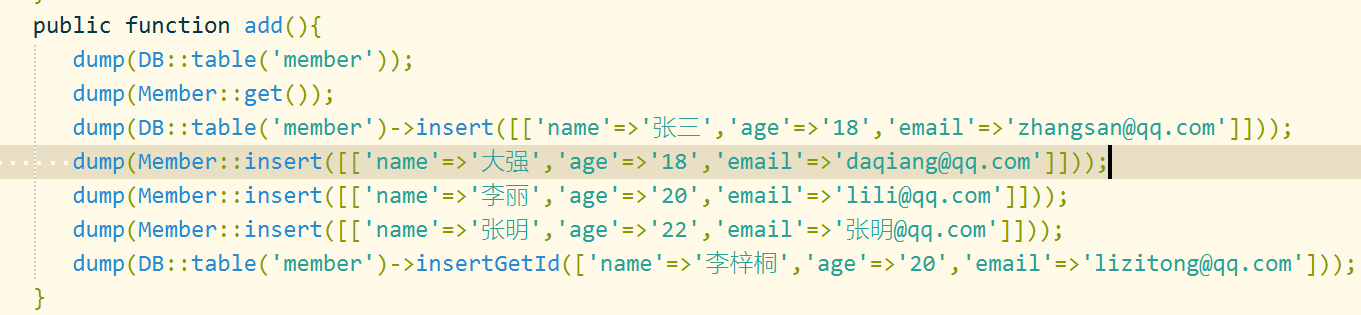注意：必须使用键值对的方式 insert与insertGetId的区别 insert能进行多条数据的插入 insertGetId只能进行单条数据的插入 3.修改操作 语法：DB::table()->where()->updata(一维数组); where的用法： obj->where(‘字段名’,‘字段符’,‘字段值’)->where(); 表示and，并且条件 obj->where(‘字段名’,‘字段符’,‘字段值’)->orwhere(); 表示or,或条件 提示：上述用法，当运算符为‘=’号的时候可以被省略 切记：修改时一定要加上条件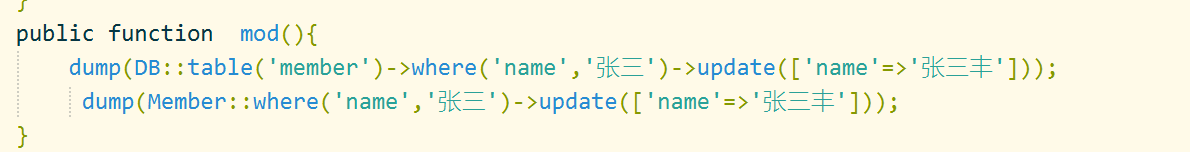4.查询操作 get表示查询所有的数据 all表示查询所有的数据，all方法前面不能有其它方法，甚至table方法也不可以(只能在模型方法中使用) value表示只获取某个字段的值 find查询某个记录 where条件原则 orderfly排序 select选择需要的字段 limit限制输出记录数 offset表示偏移量，配合limit一起使用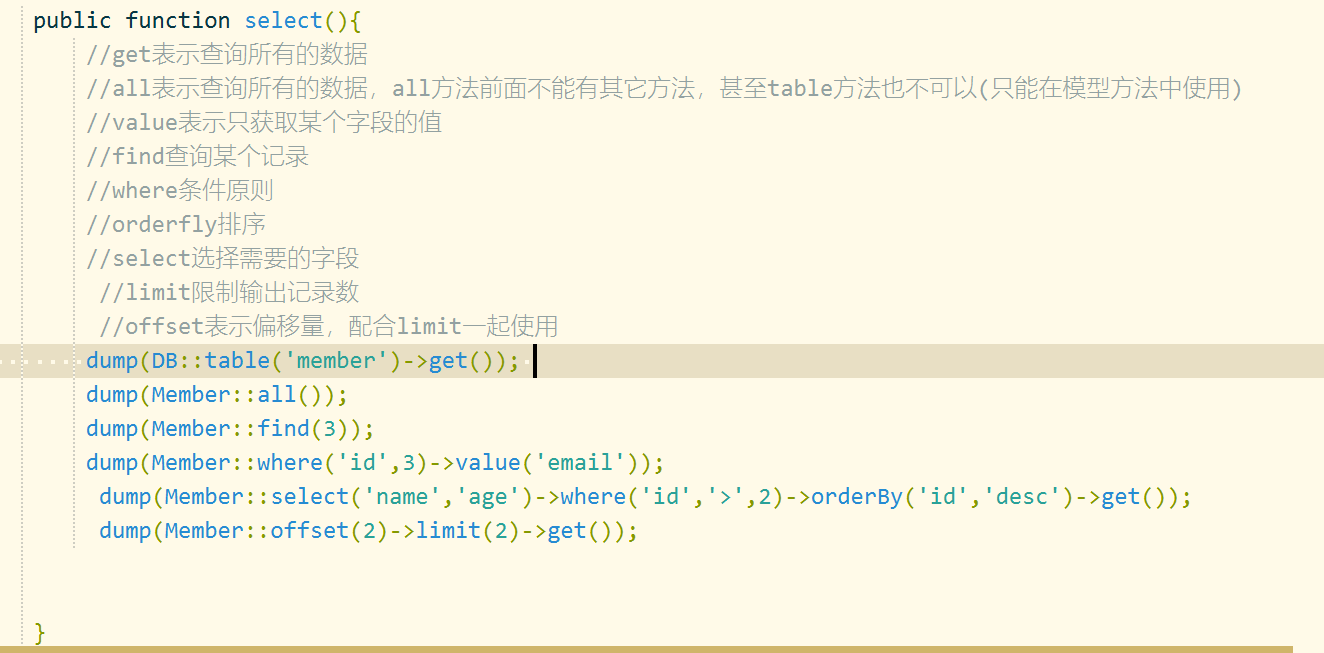offset表示的开始位置，limit表示查询多少条语句 展开全文• 主要介绍了使用Laravel中的查询构造器实现增删改查功能，本文给大家介绍的非常详细，具有一定的参考借鉴价值,需要的朋友可以参考下 • 6.3 模型基本使用（） 6.4 模型的删改 6.4.1 新增 6.4.2 更新 6.4.3 删除 6.5 模型的批量赋值 6.6 模型的软删除（逻辑删除） 6.6.1 使用软删除 6.6.2 查询软删除的数据 6.6.3 恢复软删除的数据 6.6.4 软删除后的...php sql • 首先我们需要在laravel中配置好数据库，进入laravel的目录，找到.env这个文件，在里面对数据库的主机名，数据库名，用户名，密码进行配置，配置成功之后，我们才能够对数据库进行设置，我们需要配置的就以下几项： ... • 1.数据库配置； ... post为请求方式，score/add为访问路径，ScoreController...3.在控制器里实现增删改查核心代码，取参数$request->get(‘sid’)，使用到的数据库操作有first() 查询第一条数据 save() 更新或插入数据 g
• 工作中难免要写非常多的增删改查的逻辑，Laravel的查询构造器写起来已经很舒服了，但是仍然避免不了要写大量的重复代码，比如我们要实现一个最基本的用户模块的管理功能，起码要写下面这么多接口 用户列表接口(分页)...php
• 主要介绍了Laravel使用memcached缓存对文章增删改查进行优化的方法,结合实例形式分析了Laravel框架中使用memcached缓存实现针对增删改查等操作的优化功能,需要的朋友可以参考下
• 插入数据 插入一条数据 DB::table('users')->insert([ 'name' => str_random(10), 'email' => str_random(8) . '@163.com', 'password' => bcrypt('secret') ...$userId = DB::table('u...mysql PHP • PS D:\developer\laravel> composer create-project --prefer-dist laravel/larave/ news 3 配置数据库连接 首先在 .env中配置数据库连接信息 DB_CONNECTION=mysql DB_HOST=127.0.0.1 DB_PORT=33... • laravel框架中使用orm是非常方便的操作方式，他重点实现的流程是控制器调用模型表对数据进行操作 控制器的操作代码 namespace App\Http\Controllers\Vodecontroller; use Illuminate\Http\Request; use App\... • 无刷新增删改，网上都有免费下载，所以就不设置积分了。 • 等的控制器动作是控制器postController里面所定义的方法，实现文章的各项功能的实现调用 3：定义PostController控制器方法 class PostController extends Controller{ public function index(){$posts = ...
• ：12345//save返回true false$res = new member();res-&gt;username = 'a';$res-&gt;password = 'b';dd($res-&gt;save()); 12345//返回插入数据的数组array$res = member::create(['username'=...
• Laravel5.7使用查询构造器和Eloquent ORM实现简单的增删改查操作 准备一张表： SET FOREIGN_KEY_CHECKS=0; -- ---------------------------- -- Table structure for m_pic -- ---------------------------- ...查询构造器
• Laravel自带的Eloquent ORM提供了一个美观、简单的数据库打交道的ActiveRecord实现，每张数据表都对应一个与该表进行交互的“Model模型”，模型允许你在表中进行数据查询，以及插入、更新、删除等操作。 1、每个...php
• 2021.04.22补充 实现层级树状展示 /** * 2021-04-22 * 处理省市区多维数组展示 * @param $list 数组 * @param$root 根节点 * @param $pk 主键 * @param$pid 父id * @param \$child 子集下标 * @author alan.liu * @...
• laravel框架 1.1数据库迁移 配置数据库连接 首先在.env中配置数据库连接信息 DB_CONNECTION=mysql DB_HOST=127.0.0.1 DB_PORT=3306 DB_DATABASE=news //自己的数据的名字 DB_USERNAME=root DB_PASSWORD=root 创建...
• 运用layui实现简单增删改查首先认识layui简单的效果图接下来直接上代码 首先认识layui layui（谐音：类UI) 是一款采用自身模块规范编写的前端 UI 框架，遵循原生 HTML/CSS/JS 的书写与组织形式，门槛极低，拿来即用...
• 上一节我们讲了如何使用DB门面对数据库进行原生查询，这一节我们使用另外一种方式实现对数据库的增删改查——查询构建器（Query Builder）。 获取查询构建器很简单，还是要依赖DB门面，我们使用DB门面的table...查询构造器 php
• ## layui增删改查

千次阅读 2019-09-21 14:46:52
增加 编辑 删除 搜索 引入css样式和js <link rel="stylesheet" href="css/layui.css" media="all"> <script src="layui.js"></script> <div class="layui-btn-group ...button t...
• 文章类型CRUD 1. 生成数据库 1.1 创建数据库迁移文件 ... 1.2 运行数据库迁移 php artisan migrate 2. 创建模型 ... 创建模型注意事项 表名为复数形式，模型名称为单数形式 表名如果是多个单词组...
• laravel-admin 是一个可以快速帮你构建后台管理的工具，它提供的页面组件和表单元素等功能，能帮助你使用很少的代码就实现功能完善的后台管理功能。 注意 当前版本(v1.7.*)需要安装PHP 7+和Laravel 5.5+|6.x 完成......